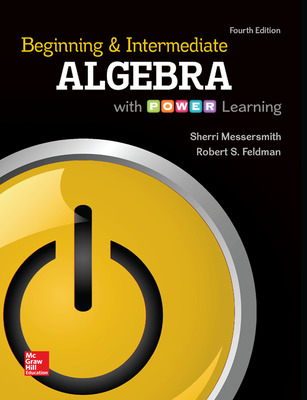# Beginning and Intermediate Algebra with P.O.W.E.R. Learning

4th Edition
By Sherri Messersmith
ISBN10: 0073512915
ISBN13: 9780073512914

## Purchase Options

Students, we’re committed to providing you with high-value course solutions backed by great service and a team that cares about your success. See tabs below to explore options and pricing. Don't forget, we accept financial aid and scholarship funds in the form of credit or debit cards.

### Hardcopy

• Bound book containing the complete text
• Full color
• Hardcover or softcover

The estimated amount of time this product will be on the market is based on a number of factors, including faculty input to instructional design and the prior revision cycle and updates to academic research-which typically results in a revision cycle ranging from every two to four years for this product. Pricing subject to change at any time.

### Program Details

Beginning & Intermediate Algebra with POWER Learning

Chapter 1 The Real Number System and Geometry

1.1 Review of Fractions

1.2 Exponents and Order of Operations

1.3 Geometry Review

1.4 Sets of Numbers and Absolute Value

1.5 Addition and Subtraction of Real Numbers

1.6 Multiplication and Division of Real Numbers

1.7 Algebraic Expressions and Properties of Real Numbers

Chapter 2 Rules of Exponents

2.1A Basic Rules of Exponents: The Product Rule and Power Rules

2.1B Basic Rules of Exponents: Combining the Rules

2.2A Integer Exponents Bases: Real-Number Bases

2.2B Integer Exponents Bases: Variable Bases

2.3 The Quotient Rule

2.4 Scientific Notation

Chapter 3 Linear Equations and Inequalities in One Variable

3.1 Solving Linear Equations Part I

3.2 Solving Linear Equations Part II

3.3 Applications of Linear Equations

3.4 Applications Involving Percents

3.5 Geometry Applications and Solving Formulas

3.6 Applications of Linear Equations to Proportions, Money Problems, and d=rt

3.7 Linear Inequalities in One Variable

3.8 Compound Inequalities in One Variable

Chapter 4 Linear Equations in Two Variables and Functions

4.1 Introduction to Linear Equations in Two Variables

4.2 Graphing by Plotting Points and Finding Intercepts

4.3 The Slope of a Line

4.4 The Slope-Intercept Form of a Line

4.5 Writing an Equation of a Line

4.6 Introduction to Functions

Chapter 5 Solving Systems of Linear Equations

5.1 Solving Systems by Graphing

5.2 Solving Systems by the Substitution Method

5.3 Solving Systems by the Elimination Method

5.4 Applications of Systems of Two Equations

5.5 Solving Systems of Three Equations and Applications

5.6 Solving Systems of Linear Equations Using Matrices

Chapter 6 Polynomials

6.1 Review of the Rules of Exponents

6.2 Addition and Subtraction of Polynomials

6.3 Multiplication of Polynomials

6.4 Division of Polynomials

Chapter 7 Factoring Polynomials

7.1 The Greatest Common Factor and Factoring by Grouping

7.2 Factoring Trinomials of the Form x2+bx+c

7.3 Factoring Trinomials of the Form ax2+bx+c (a≠1)

7.4 Factoring Special Trinomials and Binomials

7.5 Solving Quadratic Equations by Factoring

Chapter 8 Rational Expressions

8.1 Rational Expressions and Functions

8.2 Multiplying and Dividing Rational Expressions

8.3 Finding the Least Common Denominator

8.4 Adding and Subtracting Rational Expressions

8.5 Simplifying Complex Fractions

8.6 Solving Rational Equations

8.7 Applications of Rational Equations

8.8 Variation

Chapter 9 More Equations and Inequalities

9.1 Absolute Value Equations

9.2 Absolute Value Inequalities

9.3 Linear and Compound Linear Inequalities in Two Variables

Chapter 10 Radicals and Rational Exponents

10.1 Finding Roots

10.2 Rational Exponents

10.3 Simplifying Expressions Containing Square Roots

10.4 Simplifying Expressions Containing Higher Roots

10.8 Complex Numbers

11.1 Review of Solving Equations by Factoring

11.2 The Square Root Property and Completing the Square

11.5 Formulas and Applications

Chapter 12 Functions and Their Graphs

12.1 Relations and Functions

12.2 Graphs of Functions and Transformations

12.3 Quadratic Functions and Their Graphs

12.4 Applications of Quadratic Functions and Graphing Other Parabolas

12.6 The Algebra of Functions

Chapter 13 Exponential and Logarithmic Functions

13.1 Inverse Functions

13.2 Exponential Functions

13.3 Logarithmic Functions

13.4 Properties of Logarithms

13.5 Common and Natural Logarithms and Change of Base

13.6 Solving Exponential and Logarithmic Equations

Chapter 14 Conic Sections, Nonlinear Systems, and Nonlinear Inequalities

14.1 The Circle

14.2 The Ellipse and the Hyperbola

14. PIAT Putting It All Together

14.4 Second-Degree Inequalities and Systems of Inequalities

Chapter 15 Sequences and Series (online only)

15.1 Sequences and Series

15.2 Arithmetic Sequences and Series

15.3 Geometric Sequences and Series

15.4 The Binomial Theorem